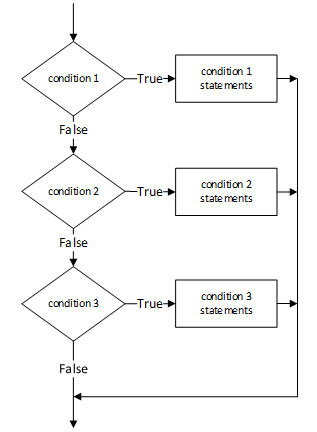## If/else If Ladder In C Language

When a programming situation requires the choice of one case from many different cases successive if statements can be tied together forming what is sometimes called an if-else-if ladder.

Syntax
```//Syntax :
if  ( condition_1 )
statement_1 ;
else if ( condition_2 )
statement_2 ;
else if ( condition_3 )
statement_3 ;
...
else if ( condition_n )
statement_n ;
else
statement_default ;```Essentially what we have here is a complete if-else statement hanging onto each else statement working from the bottom up.

For Example : Another guessing game.

CODE/PROGRAM/EXAMPLE
```void main()
{
int secret = 101, guess, count = 0 ;

printf( "\n Try and guess my secret number.\n\n" ) ;

while ( 1 )         // infinite loop until we break out of it
{
printf( "\n Make your guess: "" ) ;
scanf( "%d", &guess ) ;
count ++ ;

if (   guess < secret )
printf( "\nA little low. Try again." ) ;
else if ( guess > secret )
printf( "\nA little high. Try again.") ;
else
{
printf( "\nOk you got it and only on attempt %d.", count );
break ;
}
}
}```NOTE : Caution is advisable when coding the if-else-if ladder as it tends to be prone to error due to mismatched if-else clauses.

### Conditional Operator :- ?:

This is a special shorthand operator in C and replaces the following segment

Syntax
```if ( condition )
expr_1 ;
else
expr_2 ;```

with the more elegant

Syntax
`condition ? expr_1 : expr_2 ;`

The ?: operator is a ternary operator in that it requires three arguments. One of the advantages of the ?: operator is that it reduces simple conditions to one simple line of code which can be thrown unobtrusively into a larger section of code.

For Example :- to get the maximum of two integers, x and y, storing the larger in max.

max = x >= y ? x : y ;

The alternative to this could be as follows

Syntax
```if ( x > = y  )
max = x ;
else
max = y ;```

giving the same result but the former is a little bit more succinct.